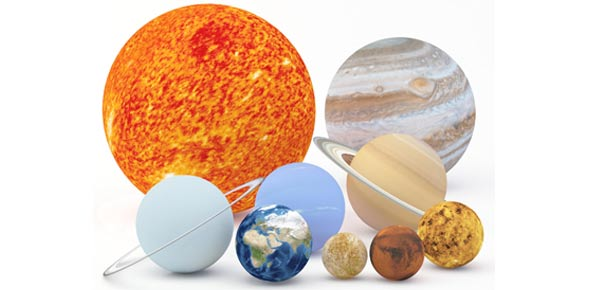# Astronomy Exam Practice Quiz Questions

8 Questions | Attempts: 246
ShareSettingsThe sun is the biggest object in our solar system, yet it is only a medium-sized star. The objects that orbit the sun are called planets where as those that don’t are moons. The review exam below is aimed at testing what you know about astronomy in less than an hour. Give it a shot!

• 1.
How are wavelength, frequency, and energy related for photons of light?
• A.

Longer wavelength means higher frequency and higher energy

• B.

Longer wavelength means lower frequency and higher energy

• C.

Longer wavelenght means lower frequency and lower energy

• D.

Longer wavelength means higher frequency and lower energy

• 2.
• A.

A hot object emits more radio waves than a cool object

• B.

A hot object emits photons with a higher average energy than a cool object

• C.

A hot object emits photons with a longer wavelength than a cool object

• D.

A hot object emits more x rays than a cool object

• 3.
From shortest to longest wavelength, which of the following correctly orders the different categories of electromagnetic radiation?
• A.

Radio, infrared, visible light, ultraviolet, x rays, gamma

• B.

Gamma rays, x rays, ultraviolet, visible light, infrared, radio

• C.

Infrared, visible light, ultraviolet, x rays, gamma rays, radio

• D.

Gamma rays, x rays, visible light, ultraviolet, infrared, radio

• 4.
The spectra of most galaxies show redshifts. This means that their spectral lines _______.
• A.

Have a higher intensity in the red part of the spectrum

• B.

Have wavelengths that are shorter than normal

• C.

Always are in the red part of the visible spectrum

• D.

Have wavelengths that are longer than normal

• 5.
Which is the closest to the temperature of the core of the Sun?
• A.

10,000 K

• B.

100,000 K

• C.

10 million K

• D.

100 million K

• 6.
Why are neutrinos so difficult to detect?
• A.

Because they have no mass

• B.

Because they rarely interact with matter

• C.

Because they move at nearly the speed of light

• D.

Because they are so rare

• E.

Because they are so small

• 7.
The most distant stars we can measure stellar parallax for are approximately
• A.

5 parsecs

• B.

100 parsecs

• C.

Halfway across the Milky Way

• D.

In the Andromeda Galaxy

• E.

10,000 parsecs

• 8.
Which of the following is true about low-mass stars compared to high-mass stars?
• A.

Low-mass stars are cooler but more luminous than high-mass stars

• B.

Low-mass stars are hotter and more luminous than high-mass stars

• C.

Low-mass stars are hotter but less luminous than high-mass stars

• D.

Low-mass stars are cooler and less luminous than high-mass stars

• E.

Low-mass stars have the same temperature and luminosity as high-mass stars

## Related TopicsBack to top
×

Wait!
Here's an interesting quiz for you.